### Solow Growth Model - Solving for Steady State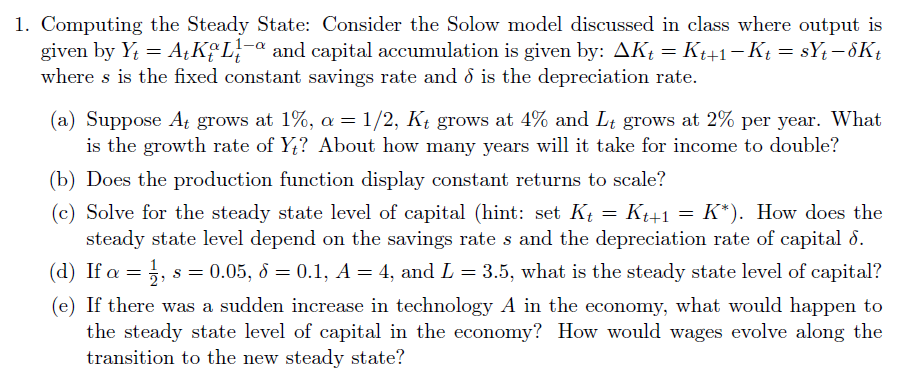SolutionsPart A - Growth Rates & Time to Double (Rule of 70)Part B - Returns to Scale - Constant or Not?Part C - Solow Growth Model - Solving for Steady StatePart D - Plugging in NumbersPart E - Using the Solow Model and Diagram (c)   See page 102, particularly page 105 (of Jones Macro Econ Crisis Update Ed)(d) is below The incantation of a steady state is the following: “A steady state is a value,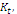, such that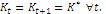This implies that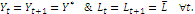"      where (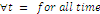). Take the two equations above, and establish the steady state. Equation One – the Capital Accumulation Equation  (aka, the Law of Motion of Capital)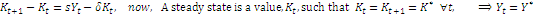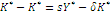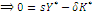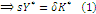,    this is our equation (1) Equation Two – The production function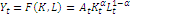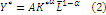,    this is our equation (2) Now – combine (1)  & (2), and solve for Capital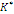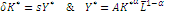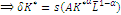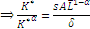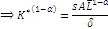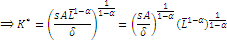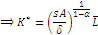You can see that the steady state level of capital (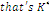) depends on all the stuff in the right-hand-side: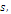the savings rate.          If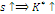, the depreciation rate,   If(d) we are just plugging numbers in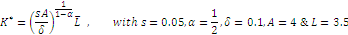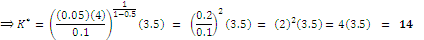(assuming I didn’t make a maths mistake along the way)